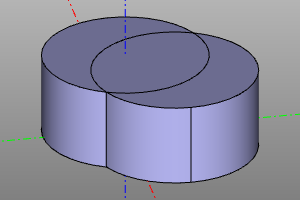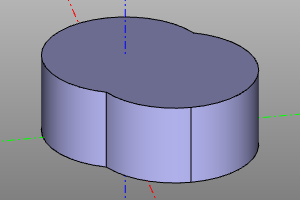# Other operations.

This section lists operations that do not fit into any other section.

## unify

This operation simplifies the geometric representation of an object by removing unnecessary edges and merging with a face.

Can be performed on 2D and 3D objects.

Signature:

``````unify(shp)
``````

Example:

``````unify(cylinder(r=10, h=10) + cylinder(r=10, h=10).move(5,5))
``````
Before After